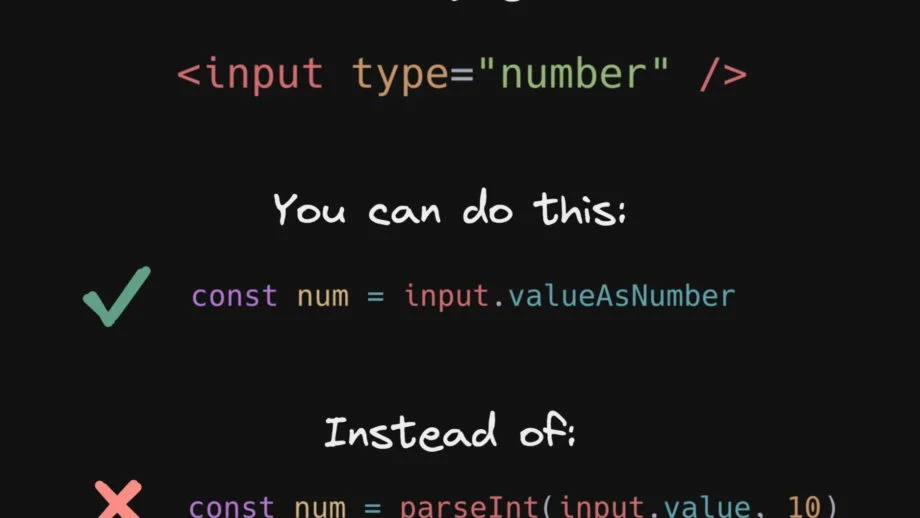# Getting the value of an input element as a number without parseInt

Share

Every once in a while, you come across something and you think, how did I not know this earlier? `valueAsNumber` is that thing for me. Whenever we need the value of an `HTMLInputElement`, we go to `e.target.value`. But getting the value of an input element as a number can be done without parseInt or parsing it.

`HTMLInputElement.valueAsNumber` is an attribute that returns a numeric value of the input field and it returns `NaN` if the value cannot be converted.

``````/*
Assuming an <input type="number" value="3.14" />
*/

console.log(input.valueAsNumber) // 3.14``````

Some things to note:

• This only works for inputs with `type="number"`
• This can also be used as a setter. So `input.valueAsNumber = 45` is valid too.
• There also is a `HTMLInputElement.valueAsDate` to get the date from an input with `type="date"`
• And there is a setter for date inputs too: `input.valueAsNumber = new Date()`